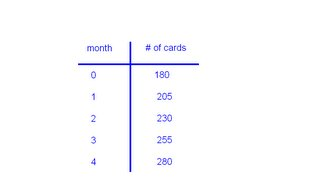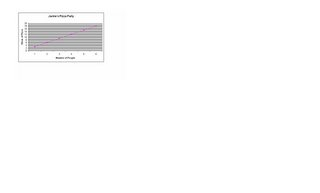## Tuesday, May 23, 2006

### My Growing Post Due Tuesday May 23rd

1. (a) Jack will have the total of 280 baseball cards at the end of the next 4 months(b) 180+25(C)= #of cards 180+25(4)=280 cards
First I started off multiplying the number of months, and that is 4, by 25, the number of cards he buys after each month. I then added his remaining 180 cards to get the total. Then he will have 280 cards at the end of the next 4 months.

(c) Jack will have the total of 1380 cards when he turns 12.
I got the answer by finding the difference between 8 and 12, and that is 4. I multiplied 4 by 12 and I got 48 because there are 48 months in 4 years. I then used the algebraic formula, 180+25(48). All that I did was change the variable. Then I multiplied 48 by 25 to give me 1200, and then I added the remaining 180 to get 1380 cards.

2.
(a) Jackie should order the total of 2 whole pizzas. She should order 2 pizzas because if you look at the chart and find 4 people there will be 12 slices and if there are 6 slices in each pizza. I divide 12 by 6 and that gave me 2 whole pizzas.(b) If two more people show up at the party, Jackie would have to order 1 more pizza, making the total 3 pizzas. She should order 3 pizzas in total becuase if you look at the chart and find 6 people there will be 18 slices. Divide 18 slices by 6 to get the total of 3 pizzas.

3. (a) Cathy will have \$200.00 dollars in her saving account. I got the answer by taking the algebraic formula of 20+15(12). I then mulitplied 12 by 15 to get 180. I then added her \$20.00 dollars to get \$200.00 dollars.
(b) If Cathy made a mistake and she had actually had \$25.00 dollars instead of \$20.00, the algebraic formula would be 25+15N= savings account total. Cathy then would have a total of \$205.00 dollars for that year.

4. (a) 3n+4n+7=2n+12

7n+7=2n+12

7n-2n(+7-7)=2n-2n(+12-7)

5n=5

n=1

(b) 8n-(4+9)=11

8n-13=11

+13 +13

8n=24

n=24/8

n=3

(c) (8-3)n+7n+8=4n+40

5n+7n+8 =4n+40

12n+8=4n+40

12n(+8-8)=4n(+40-8)

12n-4n=(4n-4n)+32

8n=32

n=4

At 5/23/2006 6:15 PM,Aria said...

Kendra, for Question 4, C; you put 4 instead of 5.

At 5/23/2006 7:10 PM,Candy said...

GREAT JOB!!!!!!!!!!! FANG

At 5/23/2006 7:23 PM,Candy said...

This comment has been removed by a blog administrator.

At 5/23/2006 8:38 PM,Aria said...

4n+405n+7n+8=

Candace, I don't think 8 - 3 is 4..

At 5/24/2006 11:05 AM,amelia_ mal said...

Kendra I think u did a great job! I didn' t even notice the mistakes. It's AMalia.

At 5/24/2006 12:01 PM,Mr. Reece said...

Great job with all of the questions. Great work with question 4 as well!

Mr. R